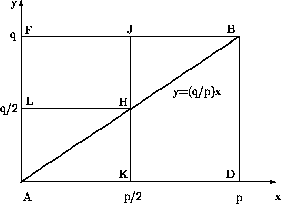# Trying to understand Gauss's Lemma (1 Viewer)

### Users Who Are Viewing This Thread (Users: 0, Guests: 1)

#### Oxymoron

Let p be an odd prime and gcd(a,p) = 1. Let n be the number of residues of the set S = {a,2a,3a,...,(p-1)/2*a} (only half the multiples) which are greater than p/2. Then (a/p) = (-1)^n.

But what if a=2 Is there an analogous lemma?

EDIT: I was right the first time

Last edited:

#### shmoe

Homework Helper
Gauss's lemma works just fine when a=2. You can use it to find (2/p) for any odd prime p.

#### Oxymoron

What I would like to do is show that

$$(2/p) = (-1)^{\frac{p-1}{2} - \left\lfloor\frac{p}{4}\right\rfloor}$$

but before I do, I would like to first understand the p odd prime case.

#### shmoe

Homework Helper
Gauss's lemma is about an alternate way of finding (a/p). If p=2, you don't need an alternate way.

#### Oxymoron

Ok, what I wrote the first time is what I meant (I promise!). Now that I have all that behind me, I would like to start to show (via Gauss's Lemma for any a) that in particular (2/p) is as I have stated above....

#### Oxymoron

Im assuming that once I can show that (2/p) = (-1)^etc... then I can show that 2 is a QR for all primes p = +-1(mod 8) and that 2 is NOT a QR for all primes p=+-3(mod 8). This is my aim, but first I would like to show the expression first.

#### Oxymoron

The only problem, I can only find the following relations in my books and online:

$$(2/p) = (-1)^{\frac{p^2-1}{8}}$$

which is not exactly what my problem wants.

#### Oxymoron

Would I be correct in assuming that

$$\frac{p-1}{2} - \left\lfloor\frac{p}{4}\right\rfloor$$

is meant to be the number of lattice points inside the triangle AHK?#### shmoe

Homework Helper
That lattice is probably coming from a proof of quadratic reciprocity, see the q on vertical axis?

You're looking at even integers S={2, 2*2, 2*3, 2*4, ..., 2*(p-1)/2} there's no need to reduce mod p here (why?). You need to count how many are greater than p/2.

Maybe you should try a few sample p's and do this manually. It should give you an idea where their exponent comes from. Remember also that p is odd, so (p-1)/2 is not an integer.

#### Oxymoron

(p-1)/2 should be an integer. p is a prime, p-1 is always even (unless p=2) so (p-1)/2 is always an integer because 2 is a divisor of every even number (unless p=2).

p exponent
3 1
5 1
7 2
11 3
13 3
17 4
19 5

Oh, and you are right That was for (p/q) = (-1)^some exp. But my point was, is the exponent related to the points in the lattice?

Last edited:

#### shmoe

Homework Helper
Oxymoron said:
p exponent
3 1
5 1
7 2
11 3
13 3
17 4
19 5
How about S? S has (p-1)/2 elements in it. To find how many are greater than p/2, you can instead find how many are less than p/2.

#### Oxymoron

p exp |S| #<p/2
3 1 1 1
5 1 2 1
7 2 3 1
11 3 5 2
13 3 6 3
17 4 8 4
19 5 9 4

Ok, Im seeing a pattern

The number of elements in S, denoted |S| will always be equal to (p-1)/2 as you said, and the number of these elements less than p/2, denoted by #<p/2 is always equal to the floor of |S|/2.

#### Oxymoron

Can I guess that the exponent is meant to resemble the number of negative least residues less than p/2?

#### Oxymoron

So this becomes a problem about showing that the number of elements in the set of negative least residues less than p.

#### shmoe

Homework Helper
Oxymoron said:
The number of elements in S, denoted |S| will always be equal to (p-1)/2 as you said, and the number of these elements less than p/2, denoted by #<p/2 is always equal to the floor of |S|/2.
Yes. Now can you write the floor of |S|/2 in a nother way? You know |S|=(p-1)/2.

Oxymoron said:
So this becomes a problem about showing that the number of elements in the set of negative least residues less than p.
I don't know what you mean by "negative least residue". In the Gauss Lemma, you take the least positive residues of the elements of S, but when p=2 they are already reduced.

#### Oxymoron

Ok so the number of elements less than p/2 in S is floor((p-1)/4).

#### shmoe

Homework Helper
Right. Now how does floor((p-1)/4) compare with floor(p/4)?

#### Oxymoron

That, I do not know. Ive been staring at it for 20 minutes.

#### shmoe

Homework Helper
Try considering the two cases p=1 or 3 mod 4 seperately, in the form p=4n+1 or +3. If p=4n+1 then what is floor(p/4) and floor((p-1)/4)?

#### Oxymoron

For p=1(mod 4) => p=4n+1 for some integer n. Then

floor((p-1)/4) = floor(n)
floor(p/4) = floor((4n+1)/4)

If p=3(mod 4) => p=4n+3 for some integer n. Then

floor((p-1)/4) = floor((4n+2)/4)
floor(p/4) = floor((4n+3)/4)

Still, I see no relationship between the two.

### The Physics Forums Way

We Value Quality
• Topics based on mainstream science
• Proper English grammar and spelling
We Value Civility
• Positive and compassionate attitudes
• Patience while debating
We Value Productivity
• Disciplined to remain on-topic
• Recognition of own weaknesses
• Solo and co-op problem solving